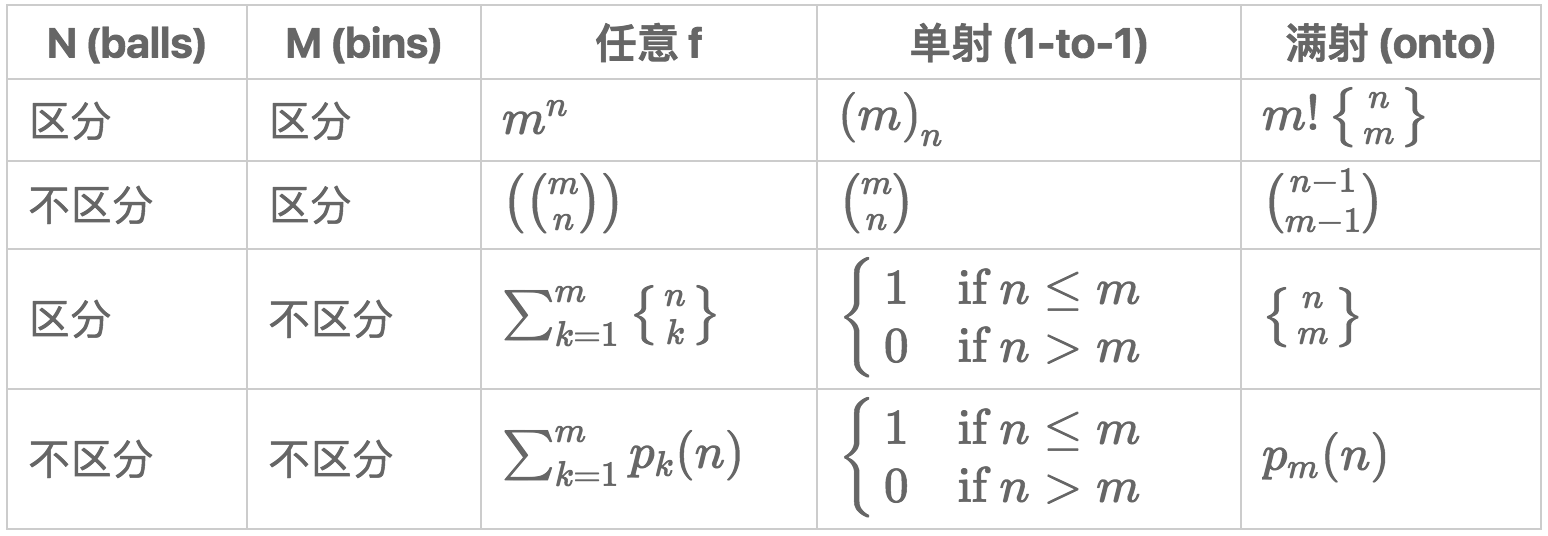## 基本计数$\left({n\choose k}\right)={n+k-1\choose k}$

## 生成函数

$G(x)=a\_0+a\_1x+a\_2x^2+\cdots$

### 生成函数的运算

Let $G(x)=\sum\_{n\ge 0}g\_nx^n$ and $F(x)=\sum\_{n\ge 0}f\_nx^n$,

\begin{align} &\text{shift:} &x^k G(x) &= \sum\_{n\ge k}g\_{n-k}x^n, &\qquad (\mbox{integer }k\ge 0)\\ &\text{addition:} & F(x)+G(x) &= \sum\_{n\ge 0} (f\_n+ g\_n)x^n\\ &\text{convolution:} &F(x)G(x) &= \sum\_{n\ge 0}\sum\_{k=0}^nf\_kg\_{n-k}x^n\\ &\text{differentiation:} &G'(x) &=\sum\_{n\ge 0}(n+1)g\_{n+1}x^n \end{align}

### 卡特兰数

$C\_n=\sum\_{k=0}^{n-1}C\_kC\_{n-1-k}$

## 容斥原理

\begin{align} \left|\bigcup\_{i=1}^nA\_i\right| &= \sum\_{I\subseteq\{1,\ldots,n\}}(-1)^{|I|-1}\left|\bigcap\_{i\in I}A\_i\right|. \end{align}

$\sum\_{I\subseteq\{1,\ldots, n\}}(-1)^{|I|}|A\_I|$

## Cayley 公式

$n$ 个顶点可以构成 $n^{n-2}$ 棵不同的树。

## 鸽巢原理

$N>mn$ 只鸽子放在 $n$ 个笼子里，存在一个笼子里有多于 $m$ 只鸽子。

## 概率方法

• 如果某个 object 被选中的概率 $p>0$，那它就存在；互补地，如果某个 object 被选中的概率 $p<1$，那它就存在。
• 如果某个随机变量的期望（均值）为 $\bar{x}$，至少存在一个取值不小于 $\bar{x}$，至少有一个取值不大于 $\bar{x}$

### Lovász 局部定理

1. 对于所有 $1\le i\le n$$\Pr[A_i]\le p$
2. 依赖图的最大度数 $d$ 满足 $ep(d+1)\le 1$

## 极值图论 *

• Mantel 定理：如果图 $G(V,E)$ 中没有三角形，则 $|E|\le\frac{n^2}{4}$
• Turán 定理：如果图 $G(V,E)$ 中没有 $r$-clique，$r\ge 2$，那么 $|E|\le\frac{r-2}{2(r-1)}n^2$
• Erdős–Stone 定理：记 $ex(n,H)$ 为：一个定点数为 $n$ 的图 $G(V,E)$ 如果要满足 $H\not\subseteq G$，最多可能有多少条边。则 $\mathrm{ex}(n,K_r)\le\frac{r-2}{2(r-1)}n^2$

## 极值集合论 *

• Erdős–Ko–Rado 定理：记 $\mathcal{F}\subseteq {X\choose k}$ ，其中$| X | = n$$n\ge 2k$ 。 如果 $\mathcal{F}$ 中的各集合两两有（非空）交集, 那么 $|\mathcal{F}|\le{n-1\choose k-1}$
• Sperner 系统：记 $\mathcal{F}\subseteq 2^X$ ，其中 $| X | = n$ 。如果 $\mathcal{F}$ 是一个 antichain，那么 $|\mathcal{F}|\le{n\choose \lfloor n/2\rfloor}$

## Ramsey 定理 *

（图论版本）定义 $k,\ell$ 为正整数。存在一个数 $R(k,\ell)$ 满足：当 $n\ge R(k,\ell)$ 时，无论怎样着色（红色和蓝色），必然存在一个红色的 $K_k$ 蓝色的 $K_\ell$

## 匹配论

### Hall 定理

• König-Egerváry 定理：二部图中，最大匹配的大小等于最小点覆盖集的大小。
• Menger 定理：在图上分开两个点至少要去掉几个点？等于这两点间不相交的路径的个数。
• Dilworth 定理：一个偏序集分成多少个 chian？等于最大 antichain 的大小。

### 最大流最小切定理

Ref.

P.S.

• 给 Ref.1 做了个备份。版权归原作者所有。